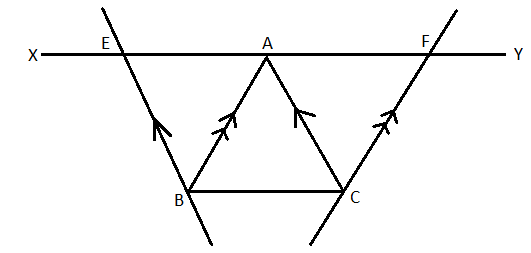QUESTION

# XY is a line parallel to side BC of a ∆ABC. If BE ll AC and CF ll AB meet XY at E and F respectively, show that:ar (∆ABE) = ar (∆ACF).

Hint: We are getting 2 parallelograms here, which are ACBE and ABCF.Since the base and height of the two parallelograms is the same, the area of the two parallelograms will also be the same.
Now, if we will subtract the area of the triangle ABC from the total area of the parallelogram, then, both the figures that are left will have the same area.